# School:Product rule for differentiation

This article combines parts of Practical:Product rule for differentiation and product rule for differentiation.

## Statement to remember

The statement of the product rule for differentiation that we will be using is:$\frac{d}{dx}[f(x)g(x)] = f'(x)g(x) + f(x)g'(x)$

where$f'(x) = \frac{d(f(x))}{dx}$ and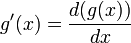$g'(x) = \frac{d(g(x))}{dx}$.

## Procedure to apply the product rule for differentiation

The product rule for differentiation is useful as a technique for differentiating functions that are expressed in the form of products of simpler functions.

### Most explicit procedure

The explicit procedure is outlined below:

1. Identify the two functions whose product is the given function. In other words, explicitly decompose the function as a product of two functions. We will here call the functions$f$ and$g$, though you may choose to give them different names.
2. Calculate the derivatives of$f$ and$g$ separately, on the side.
3. Plug into the product rule formula the expressions for the functions and their derivatives.
4. Simplify the expression thus obtained (this is optional).

Here is an example of a differentiation problem where we use this explicit procedure:

Differentiate the function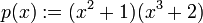$p(x) := (x^2 + 1)(x^3 + 2)$ with respect to$x$

We proceed step by step:

1. Identify the two functions: Define$f(x) = x^2 + 1$ and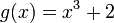$g(x) = x^3 + 2$. Then,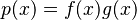$p(x)= f(x)g(x)$ by definition.
2. Calculate the derivatives: The derivative of$f(x)$ is$f'(x) = 2x$ and the derivative of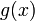$g(x)$ is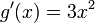$g'(x) = 3x^2$.
3. Plug into the product rule formula: We get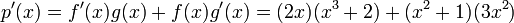$p'(x) = f'(x)g(x) + f(x)g'(x) = (2x)(x^3 + 2) + (x^2 + 1)(3x^2)$.
4. Simplify the expression obtained: We get$p'(x) = 2x^4 + 4x + 3x^4 + 3x^2 = 5x^4 + 3x^2 + 4x$.

Here is another example of a differentiation problem where we use this explicit procedure:

Differentiate the function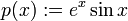$p(x) := e^x \sin x$
1. Identify the two functions: Define$f(x) = e^x$ and$g(x) = \sin x$
2. Calculate the derivatives: The derivative of$f(x)$ is$f'(x) = e^x$ and the derivative of$g(x)$ is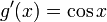$g'(x) = \cos x$.
3. Plug into the product rule formula: We get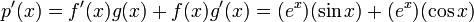$p'(x) = f'(x)g(x) + f(x)g'(x) = (e^x)(\sin x) + (e^x)(\cos x)$.
4. Simplify the expression obtained: The expression is already simplified. If we wish to collect terms, we can rewrite as$e^x(\sin x + \cos x)$.

### More inline procedure using Leibniz notation

Although the explicit procedure above is fairly clear, Step (2) of the procedure can be a waste of time in the sense of having to do the derivative calculations separately. If you are more experienced with doing differentiation quickly, you can combine Steps (2) and (3) by calculating the derivatives while plugging into the formula, rather than doing the calculations separately prior to plugging into the formula. Further, we do not need to explicitly name the functions if we use the Leibniz notation to compute the derivatives inline.

The shorter procedure is outlined below:

1. Identify the two functions being multiplied (but you don't have to give them names).
2. Plug into the formula for the product rule, using the Leibniz notation for derivatives that have not yet been computed.
3. Compute derivatives and simplify

For instance, consider the problem:

Differentiate the function$p(x) := (2x^3 + 3x - \sin x)(x^2 + \cos x - 3)$

The procedure is:

1. Identify the two functions: The functions are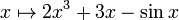$x \mapsto 2x^3 + 3x - \sin x$ and$x \mapsto x^2 + \cos x - 3$.
2. Plug into the formula for the product rule: We get: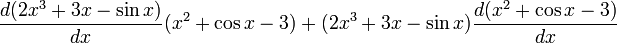$\frac{d(2x^3 + 3x - \sin x)}{dx}(x^2 + \cos x - 3) + (2x^3 + 3x - \sin x)\frac{d(x^2 + \cos x - 3)}{dx}$
3. Compute derivatives and simplify: we get:$(6x^2 + 3 - \cos x)(x^2 + \cos x - 3) + (2x^3 + 3x - \sin x)(2x - \sin x)$. The expression can be expanded and simplified if desired.

### Shortest inline procedure

If you are really experienced with doing derivatives in your head, you can shorten the procedure even further by combining Steps (2) and (3) in the previous procedure. The procedure has two steps:

1. Identify the two functions being multiplied (but you don't have to give them names).
2. Use the formula for the product rule, computing the derivatives of the functions while plugging them into the formula

For instance, going back to the example used in the beginning:

Differentiate the function$p(x) := (x^2 + 1)(x^3 + 2)$ with respect to$x$

This can be done quickly:

1. Identify the two functions: They are$x \mapsto x^2 + 1$ and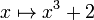$x \mapsto x^3 + 2$.
2. Use the formula for the product rule, computing the derivatives of the functions while plugging them into the formula: We get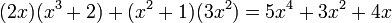$(2x)(x^3 + 2) + (x^2 + 1)(3x^2) = 5x^4 + 3x^2 + 4x$.

### Choosing between procedures

The procedures are not fundamentally different, but they differ in the degree of explicitness of the steps. Generally speaking, the following are recommended:

• If the functions being multiplied are fairly easy to differentiate mentally, use the shortest inline procedure -- this is fast and reliable.
• If the functions being multiplied are somewhat more difficult to differentiate, then choose between the other two more explicit procedures, based on whether you are more comfortable with writing large inline expressions or with doing separate work on the side.

## Error types

### Incorrect formula

A common mistake in differentiating products of functions is the freshman product rule, i.e., the false rule that the derivative of the product is the product of the derivatives. The good news is that, generally speaking, it is easy to avoid this rule once you have enough experience with the actual product rule.

### Writing only one piece of the product rule

This is an error of the incomplete task form and is harder to avoid. What happens here is that you forget to write one of the two pieces being added for the product rule, so perhaps you end up doing: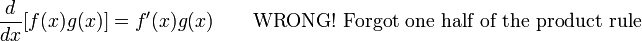$\frac{d}{dx}[f(x)g(x)] = f'(x)g(x) \qquad \mbox{WRONG! Forgot one half of the product rule}$

or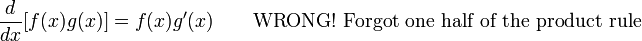$\frac{d}{dx}[f(x)g(x)] = f(x)g'(x) \qquad\mbox{WRONG! Forgot one half of the product rule}$

A slight variant involves forgetting one of the factors being multiplied: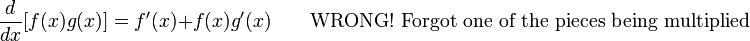$\frac{d}{dx}[f(x)g(x)] = f'(x) + f(x)g'(x) \qquad \mbox{WRONG! Forgot one of the pieces being multiplied}$

Why this error occurs: Usually, this error is common if you are trying to use the shortest inline procedure, i.e., differentiating the functions and applying the product rule simultaneously, and one of the functions being differentiated is rather tricky to differentiate, requiring a product rule or chain rule for differentiation in and of itself.

How to avoid this error:

• When the functions being differentiated are tricky to differentiate, use either the fully explicit procedure or the inline procedure with Leibniz notation. Do not try to simultaneously differentiate the pieces and use the product rule.
• After finishing a product rule problem, ask the following sanity check question: did I get a sum of two products after the application of the product rule? If the answer is no, then check your work.

## Applying the product rule for differentiation to compute values at a point

Knowledge of the values (in the sense of numerical values)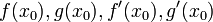$\! f(x_0), g(x_0), f'(x_0), g'(x_0)$ at a specific point$x_0$ is sufficient to compute the value of$(f \cdot g)'(x_0)$.

For instance, suppose we are given that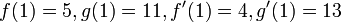$f(1) = 5, g(1) = 11, f'(1) = 4, g'(1) = 13$, we obtain that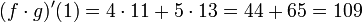$(f \cdot g)'(1) = 4 \cdot 11 + 5 \cdot 13 = 44 + 65 = 109$.

## Statement for two functions

### Statement in multiple versions

The product rule is stated in many versions:

Version type Statement
specific point, named functions Suppose$f$ and$g$ are functions of one variable, both of which are differentiable at a real number$x = x_0$. Then, the product function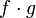$f \cdot g$, defined as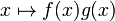$x \mapsto f(x)g(x)$ is also differentiable at$x = x_0$, and the derivative at$x_0$ is given as follows:$\! \frac{d}{dx} [f(x)g(x)]|_{x = x_0} = f'(x_0)g(x_0) + f(x_0)g'(x_0)$
or equivalently: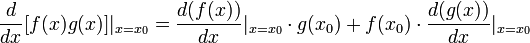$\! \frac{d}{dx} [f(x)g(x)]|_{x = x_0} = \frac{d(f(x))}{dx}|_{x=x_0} \cdot g(x_0) + f(x_0)\cdot \frac{d(g(x))}{dx}|_{x = x_0}$

generic point, named functions, point notation Suppose$f$ and$g$ are functions of one variable. Then the following is true wherever the right side expression makes sense (see concept of equality conditional to existence of one side):$\! \frac{d}{dx}[f(x)g(x)] = f'(x)g(x) + f(x)g'(x)$
generic point, named functions, point-free notation Suppose$f$ and$g$ are functions of one variable. Then, we have the following equality of functions on the domain where the right side expression makes sense (see concept of equality conditional to existence of one side):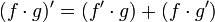$\! (f \cdot g)' = (f'\cdot g) + (f \cdot g')$
We could also write this more briefly as: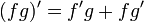$\! (fg)' = f'g + fg'$
Note that the domain of$(fg)'$ may be strictly larger than the intersection of the domains of$f'$ and$g'$, so the equality need not hold in the sense of equality as functions if we care about the domains of definition.
Pure Leibniz notation using dependent and independent variables Suppose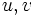$u,v$ are variables both of which are functionally dependent on$x$. Then: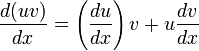$\! \frac{d(uv)}{dx} = \left(\frac{du}{dx}\right) v + u \frac{dv}{dx}$
In terms of differentials Suppose$u,v$ are both variables functionally dependent on$x$. Then,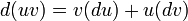$\! d(uv) = v (du) + u (dv)$.
MORE ON THE WAY THIS DEFINITION OR FACT IS PRESENTED: We first present the version that deals with a specific point (typically with a$\{ \}_0$ subscript) in the domain of the relevant functions, and then discuss the version that deals with a point that is free to move in the domain, by dropping the subscript. Why do we do this?
The purpose of the specific point version is to emphasize that the point is fixed for the duration of the definition, i.e., it does not move around while we are defining the construct or applying the fact. However, the definition or fact applies not just for a single point but for all points satisfying certain criteria, and thus we can get further interesting perspectives on it by varying the point we are considering. This is the purpose of the second, generic point version.

## Significance

### Computational feasibility significance

Each of the versions has its own computational feasibility significance:

Version type Significance
specific point, named functions This tells us that knowledge of the values (in the sense of numerical values)$\! f(x_0), g(x_0), f'(x_0), g'(x_0)$ at a specific point$x_0$ is sufficient to compute the value of$(f \cdot g)'(x_0)$. For instance, if we are given that$f(1) = 5, g(1) = 11, f'(1) = 4, g'(1) = 13$, we obtain that$(f \cdot g)'(1) = 4 \cdot 11 + 5 \cdot 13 = 44 + 65 = 109$.
A note on contrast with the (false) freshman product rule: [SHOW MORE]
generic point, named functions This tells us that knowledge of the general expressions for$f$ and$g$ and the derivatives of$f$ and$g$ is sufficient to compute the general expression for the derivative of$f \cdot g$. See the #Examples section of this page for more examples.

## Examples

### Nontrivial examples where simple alternate methods exist

Here is a simple trigonometric example:$\! f(x) := \sin x \cos x$.$\! f(x) := x \sin x$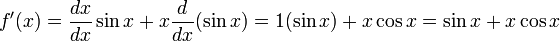$f'(x) = \frac{dx}{dx} \sin x + x \frac{d}{dx}(\sin x) = 1(\sin x) + x \cos x = \sin x + x \cos x$